# Title 40

## SECTION 89.424

### 89.424 Dilute emission sampling calculations.

§ 89.424 Dilute emission sampling calculations.

(a) The final reported emission test results are computed by use of the following formula: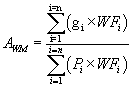Where: Awm = Weighted mass emission level (HC, CO, CO2, PM, or NOX) in g/kW-hr. gi = Mass flow in grams per hour, = grams measured during the mode divided by the sample time for the mode. WFi = Effective weighing factor. Pi = Power measured during each mode (Power set = zero for the idle mode).

(b) The mass of each pollutant for each mode for bag measurements and diesel heat exchanger system measurements is determined from the following equations:

(1) Hydrocarbon mass:

HCmass= Vmix × DensityHC × (HCconc/10 6)

(2) Oxides of nitrogen mass:

NOXmass = Vmix × DensityNO2 × KH × (NOXconc/10 6)

(3) Carbon monoxide mass:

COmass= Vmix × DensityCO × (COconc/10 6)

(4) Carbon dioxide mass:

CO2mass= Vmix × DensityCO2 × (CO2conc/102)

(c) The mass of each pollutant for the mode for flow compensated sample systems is determined from the following equations:

 

(d) Meaning of symbols:

(1) For hydrocarbon equations:

HCmass= Hydrocarbon emissions, in grams per test mode. DensityHC= Density of hydrocarbons is (.5800 kg/m 3) for #1 diesel, and (0.5746 kg/m 3) for #2 diesel, assuming an average carbon to hydrogen ratio of 1:1.93 for #1 diesel, and 1:1.80 for #2 diesel at 20 °C and 101.3 kPa pressure. HCconc= Hydrocarbon concentration of the dilute exhaust sample corrected for background, in ppm carbon equivalent (that is, equivalent propane times 3).  Where: HCe= Hydrocarbon concentration of the dilute exhaust bag sample or, for diesel heat exchanger systems, average hydrocarbon concentration of the dilute exhaust sample as calculated from the integrated HC traces, in ppm carbon equivalent. For flow compensated sample systems (HCe)i is the instantaneous concentration. HCd= Hydrocarbon concentration of the dilution air as measured, in ppm carbon equivalent.

(2) For oxides of nitrogen equations:

NOXmass = Oxides of nitrogen emissions, in grams per test mode. Density NO2= Density of oxides of nitrogen is 1.913 kg/m 3, assuming they are in the form of nitrogen dioxide, at 20 °C and 101.3 kPa pressure. NOXconc= Oxides of nitrogen concentration of the dilute exhaust sample corrected for background, in ppm:  Where: NOX.= Oxides of nitrogen concentration of the dilute exhaust bag sample as measured, in ppm. For flow compensated sample systems (NOX.)i is the instantaneous concentration. NOX.= Oxides of nitrogen concentration of the dilute air as measured, in ppm.

(3) For carbon monoxide equations:

COmass = Carbon monoxide emissions, grams per test mode. DensityCO = Density of carbon monoxide (1.164 kg/m 3 at 20 °C and 101.3 kPa pressure). COconc = Carbon monoxide concentration of the dilute exhaust sample corrected for background, water vapor, and CO2 extraction, ppm.  Where: COe = Carbon monoxide concentration of the dilute exhaust bag sample volume corrected for water vapor and carbon dioxide extraction, ppm. For flow compensated sample systems, (COe)i is the instantaneous concentration.

The following calculation assumes the carbon to hydrogen ratio of the fuel is 1:1.85. As an option the measured actual carbon to hydrogen ratio may be used:

COe=[1−0.01925CO2.−0.000323R]COem Where: COem = Carbon monoxide concentration of the dilute exhaust sample as measured, ppm. CO2. = Carbon dioxide concentration of the dilute exhaust bag sample, in percent, if measured. For flow compensated sample systems, (CO2)i is the instantaneous concentration. For cases where exhaust sampling of CO2 is not performed, the following approximation is permitted:  a = Average carbon to hydrogen ratio. M 1 = Fuel mass consumed during the mode. R = Relative humidity of the dilution air, percent. COd = Carbon monoxide concentration of the dilution air corrected for water vapor extraction, ppm. COd = (1−0.000323R)COdm Where: COdm = Carbon monoxide concentration of the dilution air sample as measured, ppm.

(Note: If a CO instrument that meets the criteria specified in 40 CFR part 1065, subpart C, is used without a sample dryer according to 40 CFR 1065.145, COem must be substituted directly for COe and COdm must be substituted directly for COd.)

(4) For carbon dioxide equation:

CO2mass = Carbon dioxide emissions, in grams per test mode. Density CO2 = Density of carbon dioxide is 1.830 kg/m 3, at 20 °C and 760 mm Hg pressure. CO2conc = Carbon dioxide concentration of the dilute exhaust sample corrected for background, in percent.  Where: CO2. = Carbon dioxide concentration of the dilution air as measured, in percent. 

(6) Measured “dry” concentrations shall be corrected to a wet basis, if not already measured on a wet basis. This section is applicable only for measurements made on dilute exhaust gas. Correction to a wet basis shall be according to the following formula:

ConcWET = KW × ConcDRY

Where: KW is determined according to the equation in paragraph (d)(6)(i) or (d)(6)(ii), of this section.

(i) For wet CO2 measurement: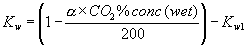(ii) For dry CO2 measurement: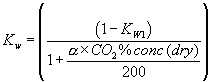(iii) For the equations in paragraph (d)(6)(i) and (d)(6)(ii) of this section, the following equation applies: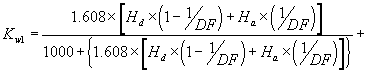Where: Ha and Hd are the grams of water per kilogram of dry air; as illustrated in the following equations: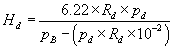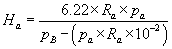(e) The final modal reported brake-specific fuel consumption (bsfc) shall be computed by use of the following formula: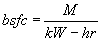Where: bsfc = brake-specific fuel consumption for a mode in grams of fuel per kilowatt-hour (kW-hr). M = mass of fuel in grams, used by the engine during a mode. kW-hr = total kilowatts integrated with respect to time for a mode.

(f) The mass of fuel for the mode is determined from mass fuel flow measurements made during the mode, or from the following equation:

 Where: M = Mass of fuel, in grams, used by the engine during the mode. Gs = Grams of carbon measured during the mode:  R2 = Grams C in fuel per gram of fuel Where: HCmass = hydrocarbon emissions, in grams for the mode CO2mass = carbon monoxide emissions, in grams for the mode CO2mass = carbon dioxide emissions, in grams for the mode α = The atomic hydrogen to carbon ratio of the fuel. [59 FR 31335, June 17, 1994. Redesignated and amended at 63 FR 56996, 57018, Oct. 23, 1998; 70 FR 40446, July 13, 2005]